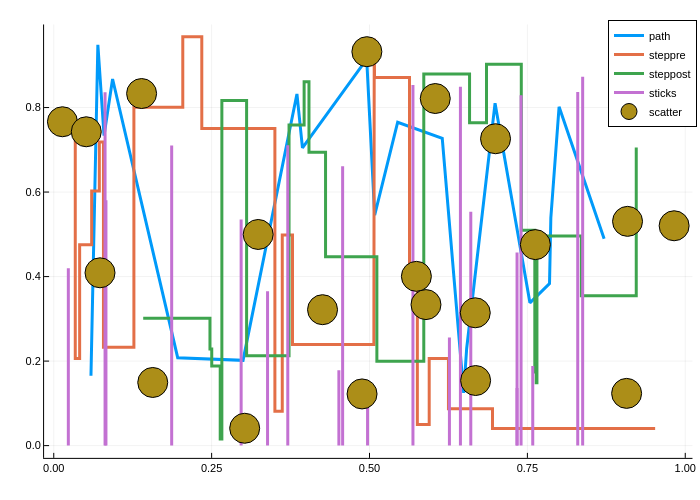# Line typesusing Plots
plotlyjs()
linetypes = [:path :steppre :steppost :sticks :scatter]
n = length(linetypes)
x = map((_->begin
sort(rand(20))
end), 1:n)
y = rand(20, n)
plot(x, y, line = (linetypes, 3), lab = map(string, linetypes), ms = 15)